Function Repository Resource:

# TriangularSets

Create a triangular set decomposition for a given list of polynomials and variables

Contributed by: Daniel Lichtblau
 ResourceFunction["TriangularSets"][polys,vars] computes a triangular decomposition of polynomials polys with respect to the ordered list of variables vars.

## Details and Options

A triangular decomposition is a type of characteristic set, as defined by Ritt and Wu.
A set of polynomials is triangular with respect to a set of variables if the last polynomial is univariate in the last variable, the penultimate one has only the last two variables, etc.
The solution set for a polynomial ideal with finitely many solutions can be represented by a list of triangular sets.
Every solution for the polynomial system is also a solution of at least one triangular set. Every solution of a triangular set is either a solution of the original system, or else causes a leading term of a set member to vanish (where the nth element is regarded as a polynomial in the nth variable, with coefficients in lesser variables).
A Gröbner basis in lexicographic order is often a triangular set. Specifically, it is a triangular set whenever the ideal is radical (has no multiple solutions), and in general position with respect to the variables (has no coordinates taking the same value in more than one solution).
ResourceFunction["TriangularSets"] uses iterated pseudoremainders, while also removing polynomial divisors common to the multiplier and remainder coefficients.
ResourceFunction["TriangularSets"] replaces powers of a polynomial with the polynomial itself. In so doing, it destroys multiplicity in a solution set.
ResourceFunction["TriangularSets"] takes a Modulus option to compute triangular sets over a prime field.

## Examples

### Basic Examples (2)

Triangularize a system of three polynomials in three variables:

 In:=Out=Check that all solutions to this system (as found by NSolve) are solutions of at least one of the triangular sets:

 In:=Out=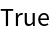### Scope (3)

TriangularSets can handle more than three variables, as illustrated on a standard benchmark known as the Trinks system:

 In:=Out=Check the size of the result in terms of number of sets and leaf count:

 In:=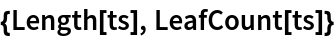Out=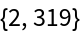Show that the solutions are all solutions of at least one triangular set:

 In:=Out=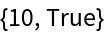Find a triangular set decomposition for a perturbation of another benchmark known as the Caprasse system:

 In:=Out=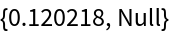Check the results:

 In:=Out=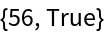Decompose the Cassou–Nouges benchmark system into triangular sets:

 In:=Out=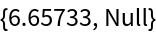Check the results:

 In:=Out=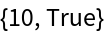### Options (3)

#### Modulus (3)

TriangularSets can be given a prime modulus:

 In:=Out=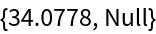Obtain the modular solutions to the original system:

 In:=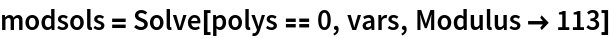Out=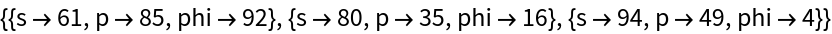The fifth triangular set contains all three solutions:

 In:=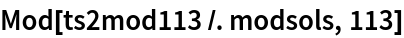Out=### Possible Issues (6)

Speed can vary considerably depending on the order of the variables:

 In:=Out=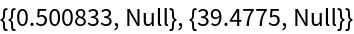The size of result can also vary considerably:

 In:=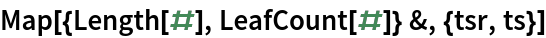Out=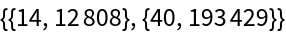Even for a seemingly small system, this function can be slow and can return a very large result. Demonstrate this on the Katsura-5 benchmark system:

 In:=Out=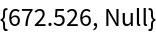Check the number of sets and the leaf count of the result:

 In:=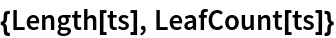Out=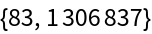Check that all solutions of this system are contained in the solution sets of the triangular sets:

 In:=Out=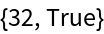## Version History

• 1.0.0 – 14 June 2021